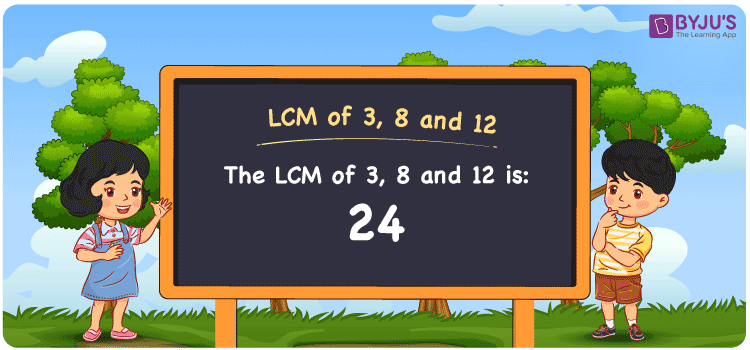# LCM of 3, 8 and 12

LCM of 3, 8 and 12 is 24. The value which is divisible evenly by the given numbers is the LCM value. Considering the common multiples of 3, 8 and 12, the least common multiples can be determined. The multiples of 3 are (3, 6, 9, 12, 15, ….), the multiples of 8 are (8, 16, 24, 32, 40, ….) and the multiples of 12 are (12, 24, 36, 48, 60, ….) respectively. Prime factorisation, division and listing of the multiples are the methods used to get the LCM

Also read: Least common multiple

## What is LCM of 3, 8 and 12?

The answer to this question is 24. The LCM of 3, 8 and 12 using various methods is shown in this article for your reference. The LCM of two non-zero integers, 3, 8 and 12, is the smallest positive integer 24 which is divisible by both 3, 8 and 12 with no remainder.## How to Find LCM of 3, 8 and 12?

LCM of 3, 8 and 12 can be found using three methods:

• Prime Factorisation
• Division method
• Listing the multiples

### LCM of 3, 8 and 12 Using Prime Factorisation Method

The prime factorisation of 3, 8 and 12, respectively, is given by:

3 = 3¹

8 = 2 x 2 x 2 = 2³

12 = 2 x 2 x 3 = 2² x 3¹

LCM (3, 8, 12) = 24

### LCM of 3, 8 and 12 Using Division Method

We’ll divide the numbers (3, 8, 12) by their prime factors to get the LCM of 3, 8 and 12 using the division method (preferably common). The LCM of 3, 8 and 12 is calculated by multiplying these divisors.

 2 3 8 12 2 3 4 6 2 3 2 3 3 3 1 3 x 1 1 1

No further division can be done.

Hence, LCM (3, 8, 12) = 24

### LCM of 3, 8 and 12 Using Listing the Multiples

To calculate the LCM of 3, 8 and 12 by listing out the common multiples, list the multiples as shown below

 Multiples of 3 Multiples of 8 Multiples of 12 3 8 12 6 16 24 9 24 36 12 32 48 15 40 60 18 48 72 21 56 84 24 64 96

LCM (3, 8, 12) = 24

## Video Lesson on Applications of LCM## LCM of 3, 8 and 12 Solved Examples

Question: What is the smallest number that can be divisible by 3, 8 and 12 exactly?

Solution:

The smallest number that can be divisible by 3, 8 and 12 exactly is the LCM.

Multiples of 3 = 3, 6, 9, 12, 15, 18, 21, 24, ….

Multiples of 8 = 8, 16, 24, 32, 40, 48, 56, …..

Multiples of 12 = 12, 24, 36, 48, 60, 72, 84, …..

Hence, the LCM is 24.

## Frequently Asked Questions on LCM of 3, 8 and 12

### What is the LCM of 3, 8 and 12?

The LCM of 3, 8 and 12 is 24.

### If the LCM of 3, 8 and 12 is 24, determine the GCF.

LCM x GCF = 3 x 8 x 12

Given

LCM of 3, 8 and 12 is 24

24 x GCF = 288

GCF = 288/24 = 12

### What are the methods used to find the LCM of 3, 8 and 12?

The methods used to find the LCM of 3, 8 and 12 are Division method, Prime Factorisation and Listing the multiples

### Show the relation between GCF and LCM of 3, 8 and 12.

The relation between GCF and LCM of 3, 8 and 12 is

GCF x LCM = 3 x 8 x 12

GCF x LCM = 288

### In 28, 24, 53, 61, what is the LCM of 3, 8 and 12?

The LCM of 3, 8 and 12 is the value which is evenly divisible by the numbers given. The number 24 satisfies this condition.